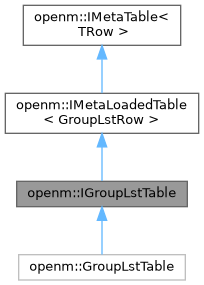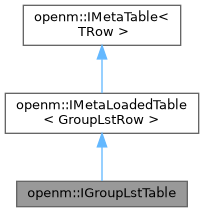OpenM++ runtime library (libopenm)
openm::IGroupLstTable Struct Referenceabstract

group_lst table public interface. More...

Inheritance diagram for openm::IGroupLstTable:[legend]
Collaboration diagram for openm::IGroupLstTable:[legend]

## Public Member Functions

virtual const GroupLstRowbyKey (int i_modelId, int i_groupId) const =0
binary search row by primary key: model id and group id, return NULL if not found.

virtual vector< GroupLstRowbyModelId (int i_modelId, bool i_isParam) const =0
get list of rows by model id and is parameter group flag.

virtual IRowBaseVecrowsRef (void)=0
get reference to list of all table rows.Public Member Functions inherited from openm::IMetaLoadedTable< GroupLstRow >
virtual const IRowBaseVecrowsCRef (void) const=0
get const reference to list of all table rows.

IRowBaseVec::difference_type rowCount (void) const
return number of rows.

vector< GroupLstRowrows (void) const
get list of loaded table rows.

const GroupLstRowfirstRow (void) const
return first table row or NULL if table is empty.

vector< GroupLstRowfindAll (RowEqual i_cmp) const
get list of rows by predicate: all rows where comparator to i_row return true.

const GroupLstRowfindFirst (RowEqual i_cmp) const
get first row by predicate or NULL if not found: first row where comparator to i_row return true.

const GroupLstRowbyIndex (IRowBaseVec::difference_type i_index) const
return row value by index or NULL if out of range.

IRowBaseVec::difference_type indexOf (RowEqual i_cmp, IRowBaseVec::difference_type i_startPos=0) const
get first row by predicate or -1 if not found: first row where comparator to i_row return true.

IRowBaseVec::size_type countOf (RowEqual i_cmp) const
count rows by predicate: where comparator to i_row return true.

## Static Public Member Functions

static IGroupLstTablecreate (IDbExec *i_dbExec, int i_modelId=0)
create new table object and load table rows sorted by primary key: model id and group id. More...

static IGroupLstTablecreate (IRowBaseVec &io_rowVec)
create new table rows by swap with supplied vector of rows.Public Types inherited from openm::IMetaLoadedTable< GroupLstRow >
typedef function< bool(const GroupLstRow &i_dbRow)> RowEqual
db table row comparator.Protected Member Functions inherited from openm::IMetaLoadedTable< GroupLstRow >
const GroupLstRowfindKey (const IRowBaseUptr &i_row) constStatic Protected Member Functions inherited from openm::IMetaLoadedTable< GroupLstRow >
load table: return vector of selected rows sorted by primary key.Static Protected Member Functions inherited from openm::IMetaTable< TRow >
static vector< TRow > rows (const IRowBaseVec &i_rowVec)
get list of table rows.

## Detailed Description

group_lst table public interface.

## ◆ create()

 IGroupLstTable * IGroupLstTable::create ( IDbExec * i_dbExec, int i_modelId = `0` )
static

create new table object and load table rows sorted by primary key: model id and group id.

if i_modelId > 0 then select only rows where model_id = i_modelId

The documentation for this struct was generated from the following files: